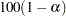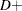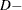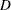PROC NPAR1WAY Statement

PROC NPAR1WAY <options> ;

The PROC NPAR1WAY statement invokes the procedure and optionally identifies the input data set or requests particular analyses. By default, the procedure uses the most recently created SAS data set and omits missing values from the analysis. If you do not specify any analysis options, PROC NPAR1WAY performs an analysis of variance (ANOVA option), tests for location differences (WILCOXON, MEDIAN, SAVAGE, and VW options), and performs empirical distribution function tests (EDF option).

Table 64.2 lists the options available in the PROC NPAR1WAY statement. Descriptions of the options follow in alphabetical order.

Table 64.2 PROC NPAR1WAY Statement Options

Options

Specify the input data set

Include missing CLASS values

Suppress all displayed output

Request analyses

Set confidence level

Suppress continuity correction

Request plots

You can specify the following options in the PROC NPAR1WAY statement.

AB

ALPHA=specifies the level of the confidence limits for location shift, which you request with the HL option. The value ofmust be between 0 and 1, and the default is 0.05. A confidence level ofproduces% confidence limits. The default of ALPHA=0.05 produces 95% confidence limits for the Hodges-Lehmann estimate.

ANOVA

requests a standard analysis of variance on the raw data.

CONOVER

requests an analysis of Conover scores. See the section Conover Scores for more information.

CORRECT=NO

suppresses the continuity correction for the Wilcoxon two-sample test and the Siegel-Tukey two-sample test. See the section Continuity Correction for more information.

D

requests the one-sided Kolmogorov-Smirnovandstatistics and their asymptotic p-values, in addition to the two-sidedstatistic produced by the EDF option for two-sample data. The D option invokes the EDF option. The statisticsandare provided by default if you request exact Kolmogorov-Smirnov statistics with the KS option in the EXACT statement for two-sample data. See the section Tests Based on the Empirical Distribution Function for details about Kolmogorov-Smirnov statistics.

DATA=SAS-data-set

names the SAS data set to be analyzed by PROC NPAR1WAY. If you omit the DATA= option, the procedure uses the most recently created SAS data set.

EDF

requests statistics based on the empirical distribution function. These include the Kolmogorov-Smirnov and Cramer-von Mises tests and, if there are only two classification levels, the Kuiper test. See the section Tests Based on the Empirical Distribution Function for more information.

The EDF option produces the Kolmogorov-Smirnovstatistic for two-sample data. You can request the one-sidedandstatistics for two-sample data with the D option.

HL

requests Hodges-Lehmann estimation of the location shift for two-sample data. The HL option provides asymptotic confidence limits for the location shift. These are sometimes known as Moses confidence limits. See the section Hodges-Lehmann Estimation of Location Shift for details. You can specify the level of the confidence limits by using the ALPHA= option. The default of ALPHA=0.5 produces 95% confidence limits for the location shift.

KLOTZ

requests an analysis of Klotz scores. See the section Klotz Scores for more information.

MEDIAN

requests an analysis of median scores. When there are two classification levels, this option produces the two-sample median test. When there are more than two samples, this option produces the multisample median test, which is also known as the Brown-Mood test. See the section Median Scores for more information.

MISSING

treats missing values of the CLASS variable as a valid class level.

MOOD

requests an analysis of Mood scores. See the section Mood Scores for more information.

NOPRINT

suppresses the display of all output. You can use the NOPRINT option when you only want to create an output data set. Note that this option temporarily disables the Output Delivery System (ODS). For more information, see Chapter 20, Using the Output Delivery System.

PLOTS < ( global-plot-options ) > < = plot-request < (plot-option) > >
PLOTS < ( global-plot-options ) > < = ( plot-request < (plot-option) > <...plot-request < (plot-option) >> )>

controls the plots that are produced through ODS Graphics. Available plots include box plots, median plots, and empirical distribution plots. See Figure 64.7, Output 64.1.2, Output 64.1.4, and Output 64.2.2 for examples of plots that PROC NPAR1WAY produces. For general information about ODS Graphics, see Chapter 21, Statistical Graphics Using ODS.

Plot-requests specify the plots to produce, plot-options apply to individual plots, and global-plot-options apply to all plots. When you specify only one plot-request, you can omit the parentheses around the request. For example:

```plots=all
plots=wilcoxonboxplot
plots=(wilcoxonboxplot edfplot)
plots(only)=(medianplot normalboxplot)
```

ODS Graphics must be enabled before requesting plots. For example:

```ods graphics on;
proc npar1way plots=wilcoxonboxplot;
variable response;
class treatment;
run;
ods graphics off;
```

For more information about enabling and disabling ODS Graphics, see the section Enabling and Disabling ODS Graphics in Chapter 21, Statistical Graphics Using ODS.

If ODS Graphics is enabled but you do not specify the PLOTS= option, PROC NPAR1WAY produces all plots that are associated with the analyses that you request. If you request a plot with the PLOTS= option but do not request the corresponding analysis, PROC NPAR1WAY automatically invokes that analysis. For example, if you specify PLOTS=CONOVERBOXPLOT but do not also specify the CONOVER option in the PROC NPAR1WAY statement, PROC NPAR1WAY produces the Conover scores analysis in addition to the box plot.

You can suppress default plots and request specific plots by using the PLOTS(ONLY)= option; PLOTS(ONLY)=(plot-requests) produces only the plots that are specified as plot-requests. You can suppress all plots with the PLOTS=NONE option. The PLOTS= option has no effect when you specify the NOPRINT option.

Global Plot Options

Global-plot-options apply to all plots produced by PROC NPAR1WAY unless they are altered by specific plot-options. You can specify the following global-plot-options in parentheses after the PLOTS option. You cannot specify both STATS and NOSTATS as global-plot-options in the same statement.

NOSTATS

suppresses the p-values that are displayed on the plots by default.

ONLY

suppresses the default plots and requests only the plots that are specified as plot-requests.

STATS

displays p-values on the plots. This is the default.

Plot Requests

The following plot-requests are available with the PLOTS= option.

ABBOXPLOT | AB

requests a box plot of Ansari-Bradley scores. This plot is associated with the Ansari-Bradley analysis, which you request with the AB option.

ALL

requests all plots that are associated with the specified analyses. This is the default if you do not specify the ONLY global-plot-option.

ANOVABOXPLOT | ANOVA

requests a box plot of the raw data. This plot is associated with the analysis of variance based on the raw data, which you request with the ANOVA option.

CONOVERBOXPLOT | CONOVER

requests a box plot of Conover scores. This plot is associated with the Conover analysis, which you request with the CONOVER option.

DATASCORESBOXPLOT | DATASCORES

requests a box plot of raw data scores. This plot is associated with the analysis that uses input data as scores, which you request with the SCORES=DATA option.

EDFPLOT | EDF

requests an empirical distribution plot. This plot is associated with the analyses based on the empirical distribution function, which you request with the EDF option.

KLOTZBOXPLOT | KLOTZ

requests a box plot of Klotz scores. This plot is associated with the Klotz analysis, which you request with the KLOTZ option.

MEDIANPLOT | MEDIAN

requests a stacked bar chart showing the frequencies above and below the overall median. This plot is associated with the median score analysis, which you request with the MEDIAN option.

MOODBOXPLOT | MOOD

requests a box plot of Mood scores. This plot is associated with the Mood analysis, which you request with the MOOD option.

NONE

suppresses all plots.

SAVAGEBOXPLOT | SAVAGE

requests a box plot of Savage scores. This plot is associated with the Savage analysis, which you request with the SAVAGE option.

STBOXPLOT | ST

requests a box plot of Siegel-Tukey scores. This plot is associated with the Siegel-Tukey analysis, which you request with the ST option.

VWBOXPLOT | VW
NORMALBOXPLOT | NORMAL

requests a box plot of Van der Waerden (normal) scores. This plot is associated with the Van der Waerden analysis, which you request with the VW or NORMAL option.

WILCOXONBOXPLOT | WILCOXON

requests a box plot of Wilcoxon scores. This plot is associated with the Wilcoxon analysis, which you request with the WILCOXON option.

Plot Options

The following plot-options are available for any plot-request. You cannot specify both STATS and NOSTATS as plot-options for the same plot. If you specify NOSTATS as a global-plot-option, specifying STATS as an individual plot-option overrides the global-plot-option for the individual plot and displays statistics on the plot.

NOSTATS

suppresses the p-values that are displayed on the plot by default.

STATS

displays p-values on the plot. This is the default.

SAVAGE

requests an analysis of Savage scores. See the section Savage Scores for more information.

SCORES=DATA

requests an analysis that uses input data as scores. This option gives you the flexibility to construct any scores for your data with the DATA step and then analyze these scores with PROC NPAR1WAY. See the section Scores for Linear Rank and One-Way ANOVA Tests for more information.

To produce the two-sample permutation test that is known as Pitman’s test, provide raw (unscored) data in the input data set and specify the SCORES=DATA option in the EXACT statement. See the section Exact Tests for more information.

ST

requests an analysis of Siegel-Tukey scores. See the section Siegel-Tukey Scores for more information.

VW | NORMAL

requests an analysis of Van der Waerden (normal) scores. See the section Van der Waerden (Normal) Scores for more information.

WILCOXON

requests an analysis of Wilcoxon scores. When there are two classification levels (samples), this option produces the Wilcoxon rank-sum test. For any number of classification levels, this option produces the Kruskal-Wallis test. See the section Wilcoxon Scores for more information.